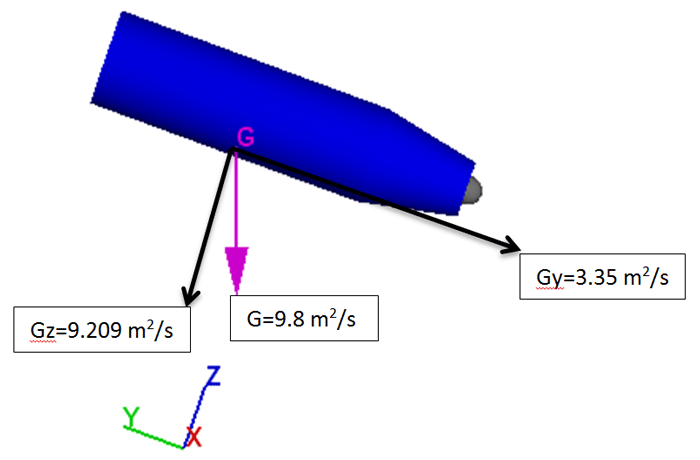In this third post of the Flow Science simulations contest blog series, I will be talking about the simulation of a Pelton turbine using FLOW-3D. This work was done by our associate in Italy, XC Engineering.

Pelton turbines are used for electricity generation in hydraulic power plants. They are suitable for operation when water energy is available at high head and low flow rate. In a Pelton turbine, the energy extracted from the kinetic energy of the water is used for the rotation of the impeller. Water, coming from an upper basin, is accelerated and ejected from the surface of the Pelton paddles. Paddle geometry is designed to absorb as much kinetic energy of the fluid as possible for the rotation of the paddle. The rotational speed of the turbine is then converted to electric power using an electricity generator with a rotor and a stator. The aim of this study is to analyze the initial transient of the turbine, where water impacts the Pelton’s paddle at around 120 m/s, providing torque and angular acceleration.

## Modelling a Pelton Turbine in FLOW-3D

The geometry used in the simulation is shown below. All geometries and data used in the simulation are realistic and in line with the real phenomena: the wheel geometry has a real shape and mass property, the fluid is water with a reasonable speed, and the nozzle contains a Doble valve (not visible here), used in real turbines to adjust the flow rate of the water.

## Moving Objects

Many kinematics are involved in this simulation, which makes FLOW-3D a very good choice for this study. The motion of an object can have all six degrees of freedom (3 rotational + 3 translational), or it can be constrained in a prescribed way. For this simulation, the Pelton turbine is allowed to have only fixed x-axis coupled rotation while staying constrained in every other direction (both rotational and translational). The other components do not move.

## Gravity and Non-Inertial Reference Frame

The figure below shows that the acceleration due to gravity is not inclined to any of the axes. This is because in the original CAD geometry, the axes are defined relative to the inlet such that inlet is parallel to the y-axis and perpendicular to the z-axis. However, for this simulation, gravity has to be in the direction shown below (pink vector) and not along any of the axes. FLOW-3D’s gravity and non-inertial reference frame model allows users to overcome such difficulties. Instead of defining a value of gravity (G) along one axis, the user can define multiple values of accelerations along multiple axes such that the net resultant is equal to G and is along the desired direction. The figure below highlights how this was done in FLOW-3D. Acceleration in the –y direction was set to 3.35 m2/s and in the –z direction to 9.209 m2/s such that the resultant is 9.8 m2/s in the desired direction.Net resultant gravity with correct magnitude and desired direction based on prescribed acceleration vectors in –y and –z directions. (Vectors are not to scale. Directions of vectors, however, are exact)

## Results

For Pelton turbines, it is known that the top efficiency is reached when the peripheral speed of the wheel is about half the speed of the water at the nozzle. For this purpose, a probe was located at the center of the nozzle to monitor the fluid speed, while another probe was attached to a paddle’s wheel, to track the peripheral speed. The two quantities are shown in the animation below.

The plots above show that by the end of the simulation, the peripheral speed is asymptotically becoming steady at more than half the velocity of the impacting fluid. Half of the impacting fluid velocity is 60m/s, but the peripheral speed reaches 75m/s by the end of the simulation. This difference (which is desirable) arises because currently the turbine is not receiving any rotational resistance from a rotor. A higher peripheral speed ensures higher kinetic energy to overcome losses in case a rotor was connected to the turbine. The final goal is to adjust, for each water velocity exiting from the nozzle, the resistance from a rotor in order to reduce the rotational speed at its maximum efficiency point and extract the energy.

Understanding the results of this study was made significantly easier by the advanced post-processing features of FlowSightTM, such as alpha transparency based on variable value, moving camera, fine tuning of light and reflections, multi-plots and multi-viewport visualization. One of the many such post-processed results is shown below to highlight the moving camera and slow motion recording of FlowSight.

FLOW-3D’s robust moving objects model backed by multi-directional acceleration prescription and state-of-the-art postprocessor, FlowSight, yields good results for this case study. In the upcoming post, I will be talking about another Flow Science contest entry based on a relatively new field of research, microfluidic circuits.Subscribe to the Blog
Privacy *